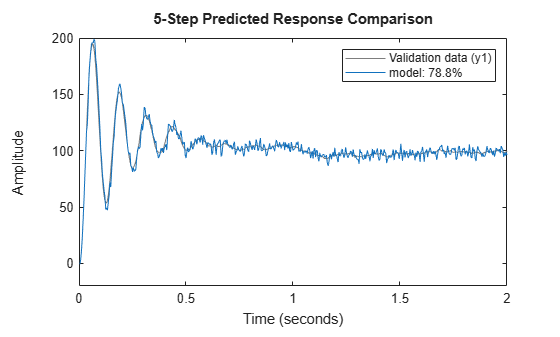Trang chủ‎ > ‎IT‎ > ‎Data Mining‎ > ‎Time Series Analysis‎ > ‎

### MATLAB - Estimate ARIMA Models

 This example shows how to estimate Autoregressive Integrated Moving Average or ARIMA models.Models of time series containing non-stationary trends (seasonality) are sometimes required. One category of such models are the ARIMA models. These models contain a fixed integrator in the noise source. Thus, if the governing equation of an ARMA model is expressed as A(q)y(t)=Ce(t), where A(q) represents the auto-regressive term and C(q) the moving average term, the corresponding model of an ARIMA model is expressed aswhere the termrepresents the discrete-time integrator. Similarly, you can formulate the equations for ARI and ARIX models.Using time-series model estimation commands ar, arx and armax you can introduce integrators into the noise source e(t). You do this by using the IntegrateNoise parameter in the estimation command.The estimation approach does not account any constant offsets in the time-series data. The ability to introduce noise integrator is not limited to time-series data alone. You can do so also for input-output models where the disturbances might be subject to seasonality. One example is the polynomial models of ARIMAX structure:See the armax reference page for examples.Estimate an ARI model for a scalar time-series with linear trend.load iddata9 z9 Ts = z9.Ts; y = cumsum(z9.y); model = ar(y,4,'ls','Ts',Ts,'IntegrateNoise', true); % 5 step ahead prediction compare(y,model,5)Estimate a multivariate time-series model such that the noise integration is present in only one of the two time series.load iddata9 z9 Ts = z9.Ts; y = z9.y; y2 = cumsum(y); % artificially construct a bivariate time series data = iddata([y, y2],[],Ts); na = [4 0; 0 4]; nc = [2;1]; model1 = armax(data, [na nc], 'IntegrateNoise',[false; true]); % Forecast the time series 100 steps into future yf = forecast(model1,data(1:100), 100); plot(data(1:100),yf)If the outputs were coupled ( na was not a diagonal matrix), the situation will be more complex and simply adding an integrator to the second noise channel will not work.